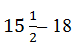Guru

# Find the number of terms in each of the following A.P. Q.5(2)

• 0

In exercise 5.2 of arithmetic progressions of class 10th. How i solve this important question of class 10th, because it is very important as regarding to board exams Find the number of terms in each of the following A.P.

Share

1. First term, a = 18

Common difference, d = a2-a1 =d = (31-36)/2 = -5/2

Let there are n terms in this A.P.

an = 205

As we know, for an A.P.,

an = a+(n−1)d

-47 = 18+(n-1)(-5/2)

-47-18 = (n-1)(-5/2)

-65 = (n-1)(-5/2)

(n-1) = -130/-5

(n-1) = 26

n = 27

Therefore, this given A.P. has 27 terms in it.

• 0